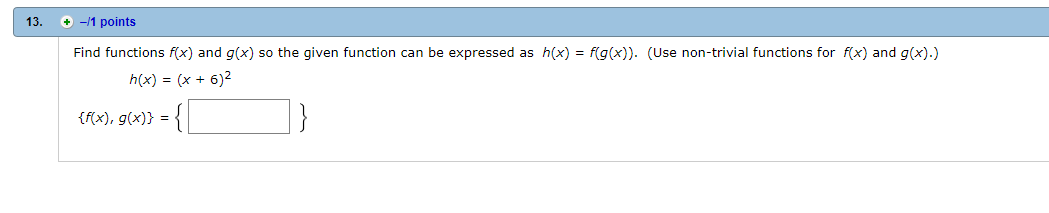# 13. + -1 points Find functions f(x) and g(x) so the given function can be expressed as h(x) = f(g(x)). (Use non-trivial functions for f(x) and g(x).) tx), g))

Question

Find function f(x) and g(x)help_outlineImage Transcriptionclose13. + -1 points Find functions f(x) and g(x) so the given function can be expressed as h(x) = f(g(x)). (Use non-trivial functions for f(x) and g(x).) tx), g)) fullscreen

### Want to see this answer and more?

Experts are waiting 24/7 to provide step-by-step solutions in as fast as 30 minutes!*